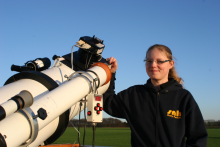# instrumental magnitudes in VPHOT

3 posts / 0 new
SNH01instrumental magnitudes in VPHOT

Hello,

I am trying to find out, how VPhot calculates the instrumental magnitudes, but I can't.

As far as I know, the instrumental magnitude m is calculated like this: m=-2.5*log(gain*counts)

But this ist not, how VPhot does it. Maybe somebody can explain me that?

Thanks
Katy

kgeinstrumental magnitudes in VPHOT

Hi Katy,

VPHOT calculate instrumental magnitudes as

m = -2.5*log10(count)

where count is the total intensity within the aperture circle pr time unit, after sky subtraction. That is, if ci is the intensity of pixel i, S is the estimated sky intensity and t the exposure time in seconds, then

count = SUM(ci - S) / t

The intensity under 'Signal Circle Statistics' on the measurement details page is SUM(ci - S), so you have to divide by exposure time before plugging it in in the first formula above to obtain the instrumental magnitude.

Geir

SNH01Hi Geir, Thank you, that

Hi Geir,

Thank you, that worked :-)

Katy